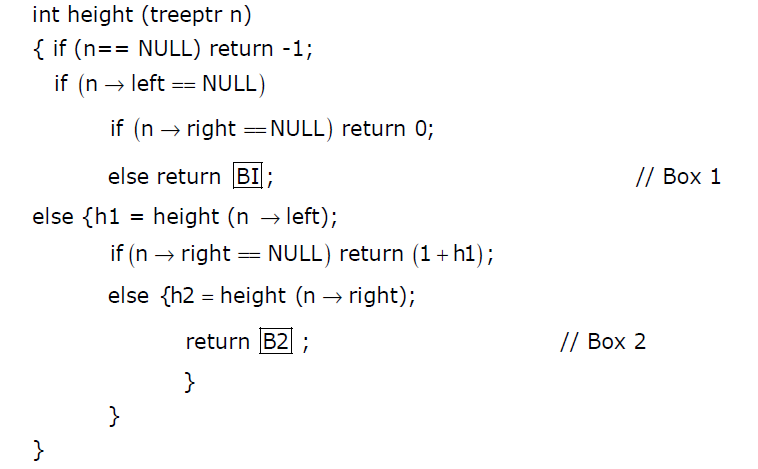# GATE | GATE CS 2012 | Question 45

The height of a tree is defined as the number of edges on the longest path in the tree. The function shown in the pseudocode below is invoked as height (root) to compute the height of a binary tree rooted at the tree pointer root.The appropriate expression for the two boxes B1 and B2 are
(A) B1 : (1 + height(n->right)), B2 : (1 + max(h1,h2))
(B) B1 : (height(n->right)), B2 : (1 + max(h1,h2))
(C) B1 : height(n->right), B2 : max(h1,h2)
(D) B1 : (1 + height(n->right)), B2 : max(h1,h2)

Explanation: The box B1 gets exected when left subtree of n is NULL and right sbtree is not NULL. In this case, height of n will be height of right subtree plus one.
The box B2 gets executed when both left and right sbtrees of n are not NULL. In this case, height of n will be max of heights of left and right sbtrees of n plus 1.

Quiz of this Question

My Personal Notes arrow_drop_up
Article Tags :

Be the First to upvote.

Please write to us at contribute@geeksforgeeks.org to report any issue with the above content.